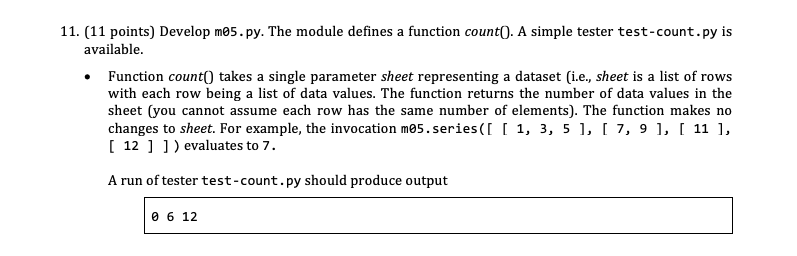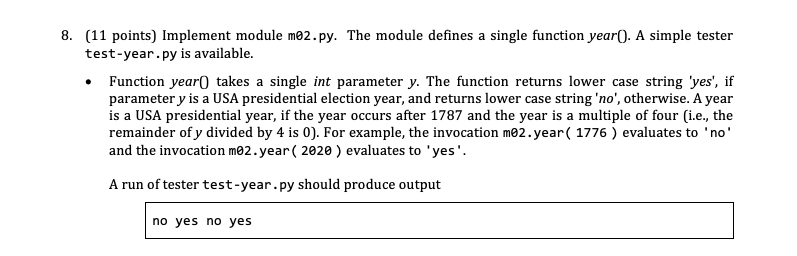## Class 32 — April 5

Part two is ending — Soon time to show mastery — Expect to do well

• Take stock

### Come on down### Accomodations

• Students with test accomodations please see me at the end of class.

### What to expect for the test

• Understanding of function and function invocation vocabulary, syntax, and semantics.
• Knowledge of `if`, `for`, and `while` statements; along with the ability to use them when problem solving.
• Ability to write functions using numbers, strings, lists, dicts, datasets, and random
• Short answer / objective questions, analysis, and implementation

### List patterns — suppose `alist`, `blist`, and `clist` are lists and that value `v` is not a list

• To analyze a list use

result = ...

for element in alist :

...

return result

• To modify a list use

n = len( alist )

for i in range( 0, n ) :

element = alist[ i ]

...

alist[ i ] = element

• Important list operations
• `alist.append( v )`
• Appends `v` to end of `alist`.
• `alist.remove( v )`
• Removes first occurence of `v` from `alist`.
• `alist.index( v )`
• Index of first occurrence of `v` in `alist`.
• `clist = alist + blist`
• Concatenates `alist` and `blist` to make `clist`.
• `v = alist.pop( )`
• Removes the last element from `alist`. The value of that former element is the return value assigned to `v`.
• `v = alist.pop( i )`
• Removes the element at index `i` from `alist`. The value of that former element is the return value assigned to `v`.
• Major gotcha

blist = alist + v # cannot add a list and a non-list

blist = alist + [ v ] # can add a list with another list

### Functions

• Functions can be invoked.
• The invocation identifies the function to be started up.
• The invocation includes the arguments. The arguments are evaluated. Copies of the values are used to initialize the parameters specified in the function definition.
• Functions must be defined. A function definition consists of a header followed by its body.
• Header: `def name ( parameters ) :`
• Body: remaining lines in function. How can you tell? They are indented. Body is also known as the statement list
• A `return` is a special statement in the body of function. When a `return` statement is reached in a function, the function stops executing its instructions; that is, the function immediately stop its execution.
• A `return` statement can optionally have a return expression following the keyword `return`. The expression is evaluated. A copy of that value is the return value of the function. The return value is said to be the value of the evaluation of the function invocation.
• All Python functions return values – if no `return` statement with a return expression is executed. The function returns `None`.
• A local variable is a variable initialized/defined inside a function definition.
• Local variables only exist inside the function definition (limited "scope")
• The parameters of a function are the variables listed with the parentheses in the function header.
• Parameters are local variables
• The arguments to a function are the values given within the parentheses of an invocation of the function.
• The Python way of handling the arguments is pass by value; that is, the arguments are evaluated to determine their values. Copies of those values are used to initialize the parameters.
• Because Python uses pass by value, the value of an argument is unchanged by a function invocation; that is, the value of an argument is the same after the invocation as it was before the invocation.
• Implication: not possible to write a swapping function in Python
• Because a parameter has the same value as its argument, if that argument is a list, the contents of that list can be modified. Such a change is called a side effect of the function. Functions with side effects generally have a return value of None.

### Warnings

• A `print()` statement in a function is not supplying a return value. It is sending a message to the program user.
• An `input()` statement is wrong to include in a function body, information comes into a function through its parameters.
• No function you will be asked to implement should have a `print()` or `input()` statement.

### Gotchas

• Nested for-loops usually wrong unless using a list-of-lists; e.g., a dataset
• Two lists? probably do not want one list inside another.

## Miscellaneous syntheses of past Q & A

### Argument versus parameter

• Defining: e.g., in `def sqrt( x )` variable `x` is the parameter. Parameters are variables defined in the parentheses of a function definition
• Using/Invoking: e.g., in `math.sqrt( 3 )` literal `3` is the argument

### `print()` versus `return`

• `print:()` display, output -- shows up in the console, not in the invoking python code
• `return`: produce, hand-back, give -- shows up in the invoking python code, not in the console
• Consider the following:

def f( x ) :

print( x )

This function displays the value of x and returns `None`

• Consider the following:

def g( x ) :

return x

This function does no display and returns the value of its parameter

### How can a function change the contents of argument memory?

• Examples

def h ( my_list, my_dictionary ) :

my_list[ index ] = ...

my_list.remove( ... )

my_list.append( ... )

my_list.pop( ... )

my_list.sort()

my_dictionary[ key ] = value

• If we do not tell you to modify a parameter, don’t. This means do not use the above statements on a list or dictionary parameter unless we ask you to update its contents
• Does that mean we can

def f( x ) :

y = x

y.pop()

No! The assignment of `x` to `y` means modifying `y` affects `x`

• Does that me we can

def f( x ) :

y = x[ : ] # or y = list( x ) or y = x.copy()

y.pop()

Yes! The assignment to `y` makes it a copy of `x` and not `x` itself

### Function `sort()` versus `sorted():`

• `x.sort()` modifies the contents of `x` to be in non-descending order and returns `None`
• `sorted( x )` creates a new list that has the elements of `x` in non-descending order, returns that new list, and does not modify `x`

### Dictionaries

• Unordered collection of key-value pairs
• Initialization examples

d = {} # empty dict

d = { 18 : 'voting', 67 : 'retirement', 'eighteen' : 18}

• Access values by key: `d[ 18 ]`
• Means the same thing as 'voting'
• Access values by key: `d[ 21 ]`
• Will be an error because 21 is not a key
• Access values by key: `d[ 'voting' ]`
• Will be an error because `'voting'` is not a key
• Access values by key: `d.get( 18 )`
• Means the same thing as `'voting'`
• Access values by key: `d.get( 21 )`
• Means the same thing as None (not an error)
• Access key by value: not easy, probably need a loop
• Collection of all keys: `d.keys()`
• Collection of all values: `d.values()`
• Indices: there are none (just keys)
• List of all keys: `list( d.keys() )`
• Set the value of a key:

d[ 100 ] = 'centarian' # makes association for key `100` to be `'centarian'`

• How do we look at the keys a `dict`

for key in d.keys():

# do something with key

• How do we look at the values of a `dict`

for value in d.values():

# do something with value

• Trying to find and change a particular entry
• By key; e.g., change key `18` to `'adult'`

• By value (find key for value 18)

found = None

for key in d.keys():

value = d[ key ]

if value == 18:

found = key.png" />